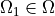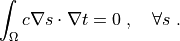# diffusion/poisson_parametric_study.py¶

Description

Poisson equation.

This example demonstrates parametric study capabilities of Application classes. In particular (written in the strong form):whereis a square domain,is a circular domain.

Now let’s see what happens ifdiameter changes.

Run:

$./simple.py <this file>  and then look in ‘output/r_omega1’ directory, try for example: $ ./postproc.py output/r_omega1/circles_in_square*.vtk


Remark: this simple case could be achieved also by definingby a time-dependent function and solve the static problem as a time-dependent problem. However, the approach below is much more general.

Findsuch that:source code

r"""
Poisson equation.

This example demonstrates parametric study capabilities of Application
classes. In particular (written in the strong form):

.. math::
c \Delta t = f \mbox{ in } \Omega,

t = 2 \mbox{ on } \Gamma_1 \;,
t = -2 \mbox{ on } \Gamma_2 \;,
f = 1 \mbox{ in } \Omega_1 \;,
f = 0 \mbox{ otherwise,}

where :math:\Omega is a square domain, :math:\Omega_1 \in \Omega is
a circular domain.

Now let's see what happens if :math:\Omega_1 diameter changes.

Run::

$./simple.py <this file> and then look in 'output/r_omega1' directory, try for example::$ ./postproc.py output/r_omega1/circles_in_square*.vtk

Remark: this simple case could be achieved also by defining
:math:\Omega_1 by a time-dependent function and solve the static
problem as a time-dependent problem. However, the approach below is much
more general.

Find :math:t such that:

.. math::
\int_{\Omega} c \nabla s \cdot \nabla t
= 0
\;, \quad \forall s \;.
"""
from __future__ import absolute_import
import os
import numpy as nm

from sfepy import data_dir
from sfepy.base.base import output

# Mesh.
filename_mesh = data_dir + '/meshes/2d/special/circles_in_square.vtk'

# Options. The value of 'parametric_hook' is the function that does the
# parametric study.
options = {
'nls' : 'newton', # Nonlinear solver
'ls' : 'ls', # Linear solver

'parametric_hook' : 'vary_omega1_size',
'output_dir' : 'output/r_omega1',
}

# Domain and subdomains.
default_diameter = 0.25
regions = {
'Omega' : 'all',
'Gamma_1' : ('vertices in (x < -0.999)', 'facet'),
'Gamma_2' : ('vertices in (x > 0.999)', 'facet'),
'Omega_1' : 'vertices by select_circ',
}

# FE field defines the FE approximation: 2_3_P1 = 2D, P1 on triangles.
field_1 = {
'name' : 'temperature',
'dtype' : 'real',
'shape' : (1,),
'region' : 'Omega',
'approx_order' : 1,
}

# Unknown and test functions (FE sense).
variables = {
't' : ('unknown field', 'temperature', 0),
's' : ('test field', 'temperature', 't'),
}

# Dirichlet boundary conditions.
ebcs = {
't1' : ('Gamma_1', {'t.0' : 2.0}),
't2' : ('Gamma_2', {'t.0' : -2.0}),
}

# Material coefficient c and source term value f.
material_1 = {
'name' : 'coef',
'values' : {
'val' : 1.0,
}
}
material_2 = {
'name' : 'source',
'values' : {
'val' : 10.0,
}
}

# Numerical quadrature and the equation.
integral_1 = {
'name' : 'i',
'order' : 2,
}

equations = {
'Poisson' : """dw_laplace.i.Omega( coef.val, s, t )
= dw_volume_lvf.i.Omega_1( source.val, s )"""
}

# Solvers.
solver_0 = {
'name' : 'ls',
'kind' : 'ls.scipy_direct',
}

solver_1 = {
'name' : 'newton',
'kind' : 'nls.newton',

'i_max'      : 1,
'eps_a'      : 1e-10,
'eps_r'      : 1.0,
'macheps'   : 1e-16,
'lin_red'    : 1e-2, # Linear system error < (eps_a * lin_red).
'ls_red'     : 0.1,
'ls_red_warp' : 0.001,
'ls_on'      : 1.1,
'ls_min'     : 1e-5,
'check'     : 0,
'delta'     : 1e-6,
}

functions = {
'select_circ': (lambda coors, domain=None:
select_circ(coors[:,0], coors[:,1], 0, default_diameter),),
}

# Functions.
def select_circ( x, y, z, diameter ):
"""Select circular subdomain of a given diameter."""
r = nm.sqrt( x**2 + y**2 )

out = nm.where(r < diameter)

n = out.shape
if n <= 3:
raise ValueError( 'too few vertices selected! (%d)' % n )

return out

def vary_omega1_size( problem ):
"""Vary size of \Omega1. Saves also the regions into options['output_dir'].

Input:
problem: Problem instance
Return:
a generator object:
1. creates new (modified) problem
2. yields the new (modified) problem and output container
3. use the output container for some logging
4. yields None (to signal next iteration to Application)
"""
from sfepy.discrete import Problem
from sfepy.solvers.ts import get_print_info

output.prefix = 'vary_omega1_size:'

diameters = nm.linspace( 0.1, 0.6, 7 ) + 0.001
ofn_trunk, output_format = problem.ofn_trunk, problem.output_format
output_dir = problem.output_dir
join = os.path.join

conf = problem.conf
cf = conf.get_raw( 'functions' )
n_digit, aux, d_format = get_print_info( len( diameters ) + 1 )
for ii, diameter in enumerate( diameters ):
output( 'iteration %d: diameter %3.2f' % (ii, diameter) )

cf['select_circ'] = (lambda coors, domain=None:
select_circ(coors[:,0], coors[:,1], 0, diameter),)
conf.edit('functions', cf)
problem = Problem.from_conf(conf)

problem.save_regions( join( output_dir, ('regions_' + d_format) % ii ),
['Omega_1'] )
region = problem.domain.regions['Omega_1']
if not region.has_cells():
raise ValueError('region %s has no cells!' % region.name)

ofn_trunk = ofn_trunk + '_' + (d_format % ii)
problem.setup_output(output_filename_trunk=ofn_trunk,
output_dir=output_dir,
output_format=output_format)

out = []
yield problem, out

out_problem, state = out[-1]

filename = join( output_dir,
('log_%s.txt' % d_format) % ii )
fd = open( filename, 'w' )
log_item = '$r(\Omega_1)$: %f\n' % diameter
fd.write( log_item )
fd.write( 'solution:\n' )
nm.savetxt(fd, state())
fd.close()

yield None